## Filters

Sort by :
Clear All
Q

A resistor has a certain color code. If one replaces the red color by green in the code, the new resistance will be :

• Option 1)

• Option 2)

• Option 3)

• Option 4)

Initially colour was Red and significant figure of red band is . Now we replace Red with green. & for green colour it is So replace with in R value. So Option 1)Option 2)Option 3)  Option 4)

In a Wheatstone bridge (see fig.) , resistances P and Qare approximately equal. When R = 400, the bridge is balanced. On interchanging P and , the valueof R, for balance, is 405. The value of X is close to:• Option 1)

401.5 ohm

• Option 2)

404.5 ohm

• Option 3)

403.5 ohm

• Option 4)

402.5 ohm

Balanced Bridge - - wherein ( Balanced condition ) No current will flow through galvanometer    Option 1)401.5 ohmOption 2)  404.5 ohmOption 3)  403.5 ohmOption 4)  402.5 ohm

In the circuit shown, the potential difference between A and B is :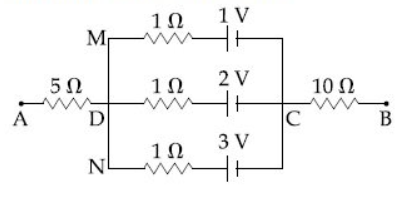• Option 1)

6 V

• Option 2)

2 V

• Option 3)

3 V

• Option 4)

1 V

In closed loop - - wherein   Option 1)6 VOption 2)2 VOption 3)3 VOption 4)1 V

In the experimental set up of metre bridge shown in the figure , the null point is obtained at a distance of 40 cm from A . If a 10 resistor is connectedin series with R1, the null point shifts by 10 cm. The resistance that should be connected in parallel with (R1 + 10 ) such that the null point shifts back to its initial position is :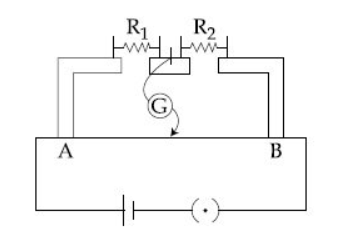• Option 1)

60

• Option 2)

40

• Option 3)

20

• Option 4)

30

Meter bridge - - wherein   Initially When connected  in series with  From (1) & (2) so  Now connect  parallel to  parallel to so  Option 1) 60 Option 2)40 Option 3)20 Option 4)30

the resistance of meter bridge AB in given figure is . With the cell of emf and rehostat resistance Rh = the null point is obtained at some point J. When the cell is replaced by another one of emf   the same null point J is found for . The emf is:• Option 1)

0.6 V

• Option 2)

0.4 V

• Option 3)

0.3 V

• Option 4)

0.5 V

Meter bridge - - wherein When  Let null point be at l cm  for     Option 1)0.6 VOption 2)0.4 VOption 3)0.3 VOption 4)  0.5 V

Two equal resistance when connected in series to a battery, consume electric power of 60W. If these resistance are now connected in parallel combination to the same battery, the electric power consumed will be:

• Option 1)

120W

• Option 2)

240 W

• Option 3)

30 W

• Option 4)

60 W

Power dissipiated in external resistance - -        Option 1)120WOption 2)240 WOption 3)30 WOption 4)60 W

The charge on a capacitor plate in a circuit, as a function of time, is shown in the figure :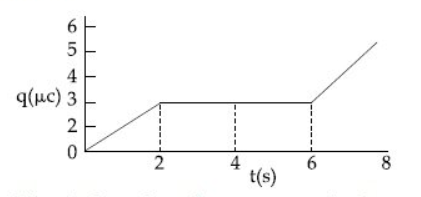What is the value of current at t = 4 s ?

• Option 1)

• Option 2)

3

• Option 3)

zero

• Option 4)

1.5

Electric current - - wherein  Instantaneous Current  tends to zero   From graph Slpoe of graph at t = 4 is zero and slpoe of graph = and current = So Option 1)2 Option 2) 3 Option 3)zeroOption 4)1.5

In the given circuit diagram, the currents, I1 = -0.3 A, I4 = 0.8 A and I5 = 0.4 A, areflowing as shown. The currents  I2 , I3  and  I6 ,respectively, are :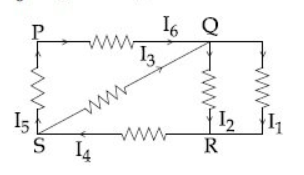• Option 1)

-0.4 A, 0.4 A, 1.1 A

• Option 2)

1.1 A -0.4 A, 0.4 A

• Option 3)

0.4 A,1.1 A ,0.4 A

• Option 4)

1.1 A ,0.4 A,0.4 A

Kirchoff's first law - - wherein   Apply Kcl at Q I6+I3 = I1+ I2   .........(1) I1 = 0.3A I4 = 0.8A I5 = 0.4A KCL at 5 I4= I5 +I3 I3 = I4 - I5     = 0.8 +0.4     =0.4A KCL at R I1+I2 = I4 I2 = I4 - I1     = 0.8 - (-0.3)     =1.1A From equation (1) I6 + I3 = I1+ I2 I6 = I1 + I2 - I3     = -0.3+1.1 -0.4     =0.4A I2 = 1.1A I3 = 0.4A I6 = 0.4A    Option 1)-0.4 A, 0.4 A, 1.1 A Option 2) 1.1...

A galvanometer , whose resistance is 50 ohm , has 25 divisions in it. When a current of 4 X 10-4 A passes through it , its needle (pointer) deflects by one division. To use this galvanometer as a voltmeter of range 2.5 V , it should be connected to a resistance of :

• Option 1)

200 ohm

• Option 2)

6200 ohm

• Option 3)

250 ohm

• Option 4)

6250 ohm

Conversion of galvanometer into voltameter - Connected a large Resistance   in series - wherein Number of divisions = N =25 Option 1)200 ohmOption 2)6200 ohmOption 3)250 ohmOption 4)6250 ohm

A current of 2 mA was passed through an unknown resistor which dissipated a power of 4.4 W. Dissipated power when an ideal power supply of 11 V is connected across it is :

• Option 1)

• Option 2)

• Option 3)

• Option 4)

Power dissipiated in external resistance - -   P = I2R Option 1)Option 2)Option 3)Option 4)

The actual value of resistance R, shown in the figure is 30  . This is measured in an experiment as shown using the standard formula , Where are the reading of the voltmeter and ammeter, respectively. If the measured value of R is 5% less, then the internal resistance of the voltmeter is: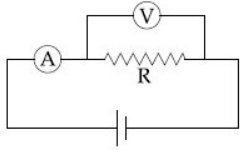• Option 1)

• Option 2)

• Option 3)

• Option 4)

Conversion of galvanometer into ammeter - Connect a low resistance in parallel to the galvanometer - wherein     Conversion of galvanometer into voltameter - Connected a large Resistance   in series - wherein  Option 1)Option 2)Option 3)Option 4)

The Wheatstone bridge shown in the figure here, get balance when the carbon resistor used as R1 has the colour code (Orange, Red, Brown) The resistor R2 and R4 are 80 and 40, respectively.

Assume that the colour code for the carbon resistor, used as R3 ,would be :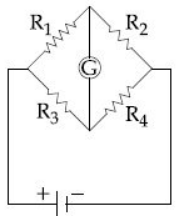• Option 1)Brown, Blue, Brown

• Option 2)

Brown, Blue, Black

• Option 3)

Red, Green, Brown

• Option 4)

Grey, Black, Brown

Colour coding of a Resistance - The carbon resistance has normally four coloured rings bands  and  . - wherein     Wheat stone Bridge - It is an arrangement of four resistances which can be used to measure one of them in terms of rest - wherein R1 = 32 X 10 = 320 R3 = 160 where 1 - brown           6 - blue           0 - brown  Option 1) Brown, Blue, BrownOption 2)Brown, Blue, BlackOption...

In the given circuit the cells have zero internal resistance. The currents (in Amperes ) Passing through resistance  and  respectively, are :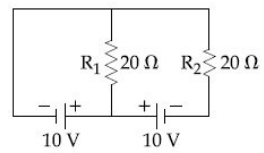• Option 1)

1,2

• Option 2)

2,2

• Option 3)

0.5,0

• Option 4)

0, 1

Ohm's Law - Current flowing through the conductor is directly proportional to the Potential difference accross two ends . - wherein unit -           Electric Resistance   for    Option 1)1,2    Option 2)2,2Option 3)0.5,0Option 4)0, 1

A 2 W carbon resistor is color-coded with green, black, red and brown respectively. The maximum current which can be passed through this resistor is :

• Option 1)

20 mA

• Option 2)

100 mA

• Option 3)

0.4 mA

• Option 4)

63 mA

To remember the sequence of colour code - B B ROY Great Britain Very Good Wife - wherein   colour code  green black red brown        Option 1)20 mAOption 2)100 mAOption 3)0.4 mAOption 4)63 mA

A uniform metallic wire has a resistance of 18 and is bent into an eqilateral triangle. Then, the resistance between any two vertices of the triangle is:

• Option 1)

4

• Option 2)

8

• Option 3)

12

• Option 4)

2

In parallel Grouping - -    Option 1)4Option 2)8 Option 3)12 Option 4)2

A potentiometer wire AB having length Land resistance 12r is joined to a cell Dof emf and internal resistance r. A cell Chaving emf and internal resistance 3r is connected. The length AJ is connected with the galvanometer as shown in the fig. shows no deflection is:• Option 1)

• Option 2)

• Option 3)

• Option 4)

Potential gradient - - wherein  internal resistance AB=L  , AJ=x So resistance of  R resistance of AJ      Option 1)Option 2)Option 3)Option 4)

In the given circuit the internal resistance of the 18V cell is negligible. If R1 = 400, R3 = 100 and R4 = 500 and the reading of an ideal voltmeter across R4 is 5V, then the value of R2 will be:• Option 1)

450

• Option 2)

300

• Option 3)

550

• Option 4)

230

Potentiometer - It is a device used to measure e.m.f of a given cell and to compare e.m.f's of cells - wherein It is also used to measure internal resistance of given cell   Current through  ,    Voltage across  =                                           =                                           = 1 V So Voltage across  Voltage across  Current through  Current through         ...

The galvanometer deflection , when key K1 is closed but K2 is open, equals (see figure). On closing K2 also and adjusting R2 to 5 , the deflection in galvanometer becomes . The resistance of the galvanometer is then , given by [ Neglect the internal resistance of battery ] :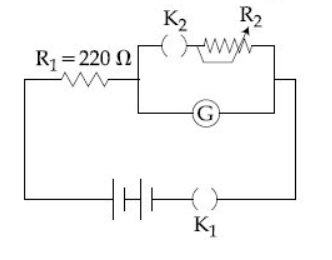• Option 1)

5

• Option 2)

12

• Option 3)

25

• Option 4)

22

Equivalent resistance - - wherein  Galvanometer resistance   Shunt resistance Case 1 Case 2 Option 1) 5 Option 2) 12 Option 3) 25 Option 4) 22

A carbon resistance has a following colour code. What is the value of resistance?• Option 1)

%

• Option 2)

%

• Option 3)

%

• Option 4)

%

To remember the sequence of colour code - B B ROY Great Britain Very Good Wife - wherein % %    Option 1)%Option 2)%Option 3)%Option 4)%

Two electric bulbs, rated at (25 W, 220 V) and (100 W,220 V ) are connected in seriees across a 220 V voltage source. If the 25 W and 100 W bulbs draw powers P1 and P2 respectively, then :

• Option 1)

P1 = 16 W , P2 = 4 W

• Option 2)

P1 = 4 W , P2 = 16 W

• Option 3)

P1 = 16 W , P2 = 9 W

• Option 4)

P1 = 9 W , P2 = 16 W

Power dissipiated in external resistance - -  &   are in series                                                                                                                                                  Option 1) P1 = 16 W , P2 = 4 WOption 2) P1 = 4 W , P2 = 16 WOption 3) P1 = 16 W , P2 = 9 WOption 4) P1 = 9 W , P2 = 16 W
Exams
Articles
Questions